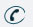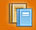781-366-0662

Subject

## Major Units of Study

Tools of Physics : Scientists use consistent and significant notation when recording and sharing experimental data. Mathematics is a tool through which scientists can organize and evaluate observations of their physical surroundings.

Topics Include : Scientific Methods, Measurement, Conversions, Vector and Scalar Quantities, Vector Addition, Vector Components
(Level 1 extensions include significant figures, accuracy and precision, and trigonometric analysis of vector quantities.)

Forces and Motion : Newton’s laws of motion and gravitation describe and predict the motion of most objects.

Topics Include : Linear Motion, Speed, Velocity, and Acceleration, Free Fall, Projectile Motion, Graphs of Motion, Newton’s Laws, Mass and Inertia, Equilibrium, Friction, Newton’s Law of Universal Gravitation, The Inverse Square Law
(Level 1 extensions include circular motion, angular and tangential displacement, velocity and acceleration, motion on an incline, torque, and multi-step mathematical analysis of motion problems.)

Conservation of Energy and Momentum : The laws of conservation of energy and momentum provide alternate approaches to predict and describe the movement of objects.

Topics Include : Linear Momentum, Elastic and Inelastic Collisions, Impulse, Conservation of Momentum, Work, Power, Mechanical Energy: Potential and Kinetic, Conservation of Energy, Simple Machines
(Extensions for Level 1 include multidimensional momentum problems and the work-energy theorem for net force.)

Vibrations and Waves : Waves carry energy from place to place without the transfer of matter. Oscillating electric or magnetic fields can generate electromagnetic waves over a wide spectrum.

Topics Include : Periodic Motion, Transverse and Longitudinal Waves, Mechanical and Electromagnetic Waves, Doppler Shift, Standing Waves and Wave Interference, Sound, Light, Geometric Optics
(Extensions for Level 1 include Hooke’s Law, simple harmonic motion, sinusoidal motion, sound intensity and the human ear, sound pitch and quality, and Snell’s Law.)

Electromagnetism : Stationary and moving charged particles result in the phenomena known as electricity and magnetism.

Topics Include : Electrostatics, Electric Fields and Potential, Electric Current, Electric Circuits, Magnetism
(Extensions for Level 1 include analysis of multi-step electricity and magnetism problems.)Subject Classes